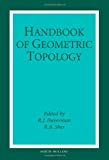• Author(s) R.B. Sher / R.J. Daverman
• Edition
• Published20th December 2001
• PublisherNorth Holland
• ISBN9780444824325

Topics in transformation groups (A. Adem and J.F .Davis).

Piecewise linear topology (J.L. Bryant).

Infinite dimens ional topology and shape theory (A. Chigogidze).

Nonposit ive curvature and reflection groups (M.W. Davis).

Nielsen fixed point theory (R. Geoghegan).

Mapping class groups (N.V. Ivanov).

Seifert manifolds (Kyung Bai Lee and F. Ra ymond).

Quantum invariants of 3-manifolds and CW-complexe s (W. Lueck).

Hyperbolic manifolds (J.G .Ratcliffe).
< BR id='LF'>Flows with knotted closed orbits (J. Franks and M.C. Sullivan ).

Heegaard splittings of compact 3-manifolds (M. Scharle mann).

Representations of 3-manifold groups (P.B. Schalen ).

Homology manifolds (S. Weinberger).

R-t rees in topology, geometry, and group theory (F. Bonathon).

Dehn surgery on knots (S. Boyer).

Geometric group theo ry (J. Cannon).

Cohomological dimension theory (J. Dydak) .

Metric spaces of curvature greater than or equal to k (

# Handbook of Geometric Topology

##### Print on Demand

Leaves '25-30' days after printing

\$309.75 \$361.00 Save \$51.25
##### Get it instantly

Zookal account needed

\$295.92 \$302.50 Save \$6.58
• Author(s) R.B. Sher / R.J. Daverman
• Edition
• Published20th December 2001
• PublisherNorth Holland
• ISBN9780444824325

Topics in transformation groups (A. Adem and J.F .Davis).

Piecewise linear topology (J.L. Bryant).

Infinite dimens ional topology and shape theory (A. Chigogidze).

Nonposit ive curvature and reflection groups (M.W. Davis).

Nielsen fixed point theory (R. Geoghegan).

Mapping class groups (N.V. Ivanov).

Seifert manifolds (Kyung Bai Lee and F. Ra ymond).

Quantum invariants of 3-manifolds and CW-complexe s (W. Lueck).

Hyperbolic manifolds (J.G .Ratcliffe).
< BR id='LF'>Flows with knotted closed orbits (J. Franks and M.C. Sullivan ).

Heegaard splittings of compact 3-manifolds (M. Scharle mann).

Representations of 3-manifold groups (P.B. Schalen ).

Homology manifolds (S. Weinberger).

R-t rees in topology, geometry, and group theory (F. Bonathon).

Dehn surgery on knots (S. Boyer).

Geometric group theo ry (J. Cannon).

Cohomological dimension theory (J. Dydak) .

Metric spaces of curvature greater than or equal to k (